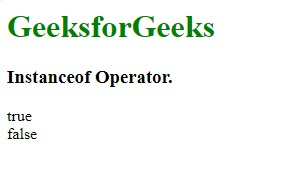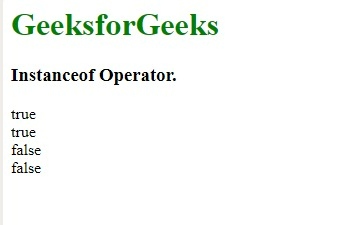GFG App
Open AppBrowser
Continue

# JavaScript Instanceof Operator

The instanceof operator in JavaScript is used to check the type of an object at run time. It returns a boolean value if true then it indicates that the object is an instance of a particular class and if false then it is not.

Syntax:

`var gfg = objectName instanceof objectType`

Parameters: This method accepts a single parameter.

• objectName: States the name of the Object.

Return Value: This method returns a boolean value if true then it indicates that the object is an instance of a particular class and if false then it is not.

Example 1: Below is the example of the Instanceof Operator.

## html

 `<``h1` `style``=``"color:green"``>` `    ``GeeksforGeeks` `` `<``h3``>` `    ``Instanceof Operator.` ``   `<``p` `id``=``"GFG"``>`   `<``script``>` `    ``var a = ["Geeks", "for", "Geeks"];` `    `  `    ``document.getElementById("GFG").innerHTML =` `        ``(a instanceof Array) + "<``br``>" +` `        ``(a instanceof Number);` ``

Output:JavaScript Instanceof Operator

Example 2:

## html

 `<``h1` `style``=``"color:green"``>` `    ``GeeksforGeeks` `` `<``h3``>` `    ``Instanceof Operator.` ``   `<``p` `id``=``"GFG"``>`   `<``script``>` `    ``var fruits = ["Apple", "Mango", "Banana"];` `    `  `    ``document.getElementById("GFG").innerHTML =` `        ``(fruits instanceof Array) + "<``br``>" +` `        ``(fruits instanceof Object) + "<``br``>" +` `        ``(fruits instanceof String) + "<``br``>" +` `        ``(fruits instanceof Number);` ``

Output:JavaScript Instanceof Operator

Example 3: Demonstrating that String and Date objects are also a type of Object (derived from Object).

## Javascript

 ``

Output:

```true
false
true
true
true
false```

We have a complete list of Javascript Operators, to check those please go through the Javascript Operators Complete Reference article.

Supported Browsers: